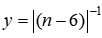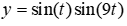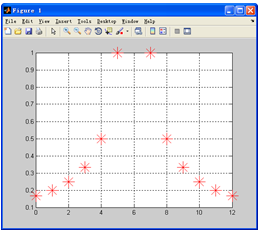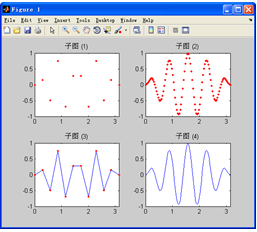matlab 平滑曲线连接_MATLAB数据可视化

(1)交互性：用户可以方便地以交互的方式管理和开发数据。

(2)多维性：可以看到表示对象或事件的数据的多个属性或变量，而数据可以按其每一维的值，将其分类、排序、组合和显示。

(3)可视化：数据可以用图像、曲线、二维图形、三维图形和动画等来显示，并可对其模式和相互关系进行可视化分析。

7.1  绘图的基本知识

7.1.1  离散数据和离散函数的可视化

【例7-1】  用图形表示离散函数Ex_7_1.m

n=0:12;

y=1./abs(n-6);                              %  准备离散点数据

plot(n,y,'r*','MarkerSize',20)           %  绘图

grid on

7.1.2  连续函数的可视化

【例7-2】  用图形表示连续调制波形Ex_7_2.m

t1=(0:11)/11*pi;              %  自变量

y1=sin(t1).*sin(9*t1);        %  对应的函数值

t2=(0:100)/100*pi;

y2=sin(t2).*sin(9*t2);

%  以下为绘图，将几个图作为子图放在一起便于比较

subplot(2,2,1),plot(t1,y1,'r.'),axis([0,pi,-1,1]),title('子图 (1)')

subplot(2,2,2),plot(t2,y2,'r.'),axis([0,pi,-1,1]),title('子图 (2)')

subplot(2,2,3),plot(t1,y1,t1,y1,'r.')

axis([0,pi,-1,1]),title('子图 (3)')

subplot(2,2,4),plot(t2,y2)

axis([0,pi,-1,1]),title('子图 (4)')7.1.3  可视化的一般步骤

 步    骤 典型命令 1．数据准备选定所要绘图的范围产生自变量采样向量计算相应的函数值向量 t=pi*(0:100)/100y=sin(t).*sin(9*t); 2．选定图形窗口及子图位置默认打开Figure. 1，或当前窗口，或当前子图。可以用命令指定图形窗口和子图位置 figure(1)subplot(2,2,3) 3．调用绘图命令(可以包括线型、色彩、数据点型) plot(t,y,‘b-’)      %   用蓝色实线绘图 4．设置轴的范围与刻度、坐标网格 axis([0,pi,-1,1])    %  设置轴的范围grid on           %  画坐标网格 5．图形注释：图名、坐标名、图例、文字说明等 title(‘figure’)        %   图名xlabel(‘t’); ylabel(‘y’)  %  轴名legend(‘sin(t)’, ‘sin(t)。*sin(9*t)’)  %  图例text(2,0.5,‘y=sin(t).*sin(9*t)’)% 文字说明 6．图形的精细修饰利用对象属性值设置，利用图形窗口工具栏设置 set(h,‘MarkerSize’,10)  %  设置数据点大小 7．图形的导出与打印 %  采用图形窗口菜单操作12-02
03-1003-163088
12-031万+
03-24240
12-221137
11-203268
11-22252
03-16120
12-20564
07-131998
04-17576
10-09545
02-2476
08-024616
10-29324
12-02
04-211万+
03-172071点击重新获取扫码支付余额充值